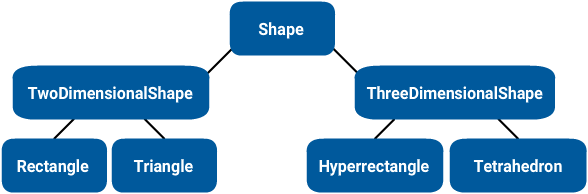# C++ Inheritance - 1 - Base and Derived Classes## What is Inheritance?

Inheritance is a form of software reuse in which a class can use another class’s methods and members and modify them. A base class can inherit the members of a derived class, then the base class can customize or enhance the derived class. In Java and C#, the base class is called superclass and the derived class is called subclass. Inheritance is represented by the is-a relationship. You can say that a derived class Rectangle is a Shape, where Shape is the base class in this scenario.

## Base and Derived Classes

Base classes are more general while derived classes are more specific. This is easy to understand if we think of Shape as a base class and Rectangle or Triangle as a derived class. Relationships of inheritance form class hierarchies, as shown in the image below. From top to bottom, you can see that Shape is a TwoDimensionalShape or is a ThreeDimensionalShape. TwoDimensionalShape in turn is a Rectangle or is a Triangle. From bottom to top, Rectangle is a TwoDimensionalShape, which in turn is a Shape.To specify that class Rectangle is derived from Shape in C++, you can type the Rectangle’s definition as:

``class Rectangle : public Shape``

This is an example of public inheritance which is the most commonly used form. You could also define it as private or protected inheritance, which I’ll discuss in another article.

## An example of inheritance

Let me demonstrate inheritance with an example. I’m going to skip the ThreeDimensionalShape class here and just assume that all shapes are two dimensional in this example. Observe the two classes below, Rectangle and Triangle. They basically share the same code, with one minor exception in getArea() where Rectangle multiplies width and height, while triangle multiplies width and height and then divides the result with 2:

``````class Rectangle {
public:

void setWidth(double width) {
this->width = width;
}

void setHeight(double height) {
this->height = height;
}

double getWidth() const {
return width;
}

double getHeight() const {
return height;
}

double getArea() {
return getWidth()* getHeight();
}

private:
double width;
double height;
};

class Triangle {
public:

void setWidth(double width) {
this->width = width;
}

void setHeight(double height) {
this->height = height;
}

double getWidth() const {
return width;
}

double getHeight() const {
return height;
}

double getArea() {
return (getWidth() * getHeight()) / 2;
}

private:
double width;
double height;
};``````

This is a classic case where the use of inheritance might be a good idea. So how should we proceed to make that happen? Let’s create a new class called Shape which will be the base class, Rectangle and Triangle will be derived by the class Shape.

In the code below, the getters and setters are moved to Shape, along with the members width and height. The only methods we don’t change in Rectangle and Triangle is getArea() since they are implemented differently. Notice the colon (:) in Rectangle and Triangle, it indicates inheritance. The public keyword just before the colon indicates the type of inheritance.

``````#include <iostream>

// Base class
class Shape {
public:
void setWidth(int width) {
this->width = width;
}

void setHeight(int height) {
this->height = height;
}

double getWidth() {
return width;
}

double getHeight() {
return height;
}

private:
double width;
double height;
};

// Derived class
class Rectangle : public Shape {
public:
double getArea() {
return getWidth() * getHeight();
}
};

// Another derived class
class Triangle : public Shape {
public:
double getArea() {
return (getWidth() * getHeight()) / 2;
}
};``````

Now we can create instances of Rectangle and Triangle and use the methods that are defined in Shape.

``````int main() {
Rectangle rect;
rect.setWidth(5);
rect.setHeight(5);

Triangle tri;
tri.setWidth(10);
tri.setHeight(10);

std::cout << "The rectangles area is " << rect.getArea() << "\n";
std::cout << "The triangles area is " << tri.getArea() << "\n";
}``````

Author### Articles with similar tags